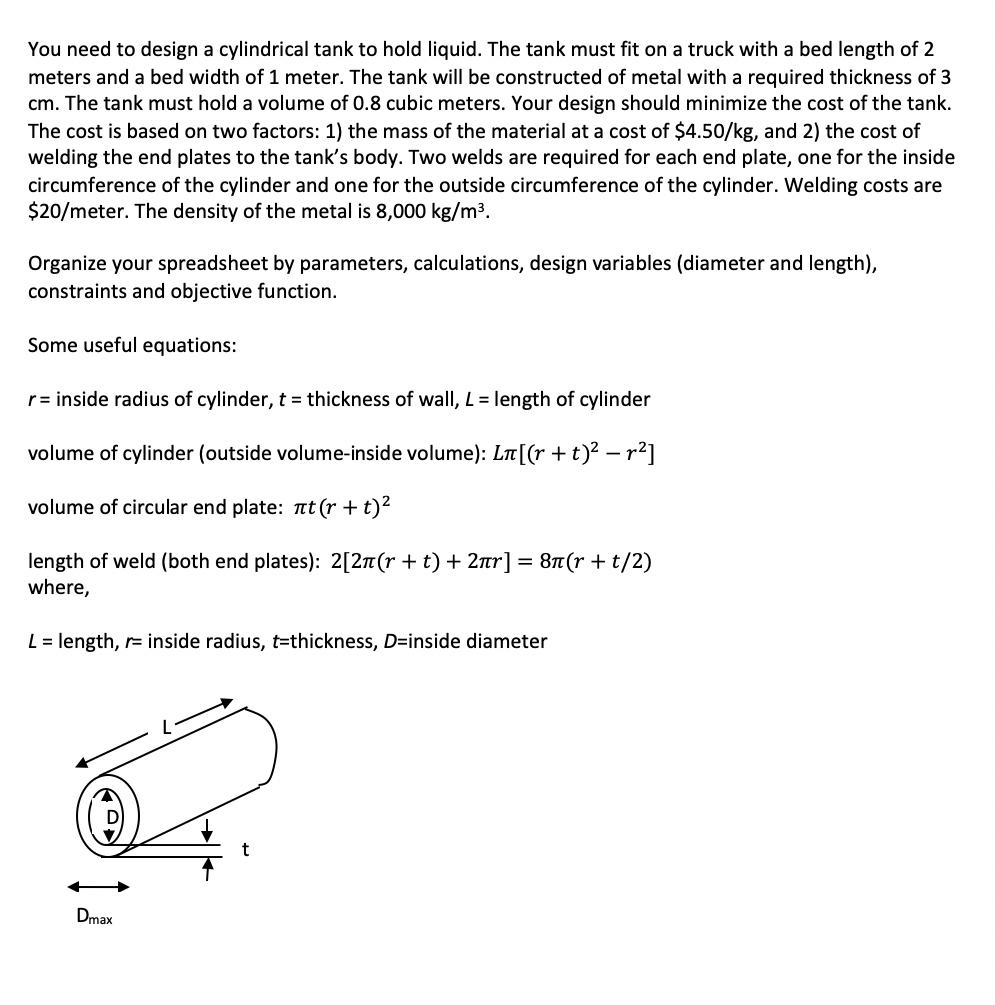# (Solved) : Use Excel Solver Ad Must Done Using Excel Please Show Step Functions Used Excel Q42721939 . . .

MUST BE DONE USING EXCEL

PLEASE SHOW EACH STEP AND ALL FUNCTIONS USED IN EXCELYou need to design a cylindrical tank to hold liquid. The tank must fit on a truck with a bed length of 2 meters and a bed width of 1 meter. The tank will be constructed of metal with a required thickness of 3 cm. The tank must hold a volume of 0.8 cubic meters. Your design should minimize the cost of the tank. The cost is based on two factors: 1) the mass of the material at a cost of \$4.50/kg, and 2) the cost of welding the end plates to the tank’s body. Two welds are required for each end plate, one for the inside circumference of the cylinder and one for the outside circumference of the cylinder. Welding costs are \$20/meter. The density of the metal is 8,000 kg/m3. Organize your spreadsheet by parameters, calculations, design variables (diameter and length), constraints and objective function. Some useful equations: r= inside radius of cylinder, t = thickness of wall, L = length of cylinder volume of cylinder (outside volume-inside volume): LT[(r+t)2 – r2] volume of circular end plate: at(r + t)2 length of weld (both end plates): 2[21(r + t) + 2nr] = 8(r + t/2) where, L = length, r= inside radius, t=thickness, D=inside diameter – Dmax Show transcribed image text You need to design a cylindrical tank to hold liquid. The tank must fit on a truck with a bed length of 2 meters and a bed width of 1 meter. The tank will be constructed of metal with a required thickness of 3 cm. The tank must hold a volume of 0.8 cubic meters. Your design should minimize the cost of the tank. The cost is based on two factors: 1) the mass of the material at a cost of \$4.50/kg, and 2) the cost of welding the end plates to the tank’s body. Two welds are required for each end plate, one for the inside circumference of the cylinder and one for the outside circumference of the cylinder. Welding costs are \$20/meter. The density of the metal is 8,000 kg/m3. Organize your spreadsheet by parameters, calculations, design variables (diameter and length), constraints and objective function. Some useful equations: r= inside radius of cylinder, t = thickness of wall, L = length of cylinder volume of cylinder (outside volume-inside volume): LT[(r+t)2 – r2] volume of circular end plate: at(r + t)2 length of weld (both end plates): 2[21(r + t) + 2nr] = 8(r + t/2) where, L = length, r= inside radius, t=thickness, D=inside diameter – Dmax

Answer to Use Excel Solver ad on… MUST BE DONE USING EXCEL PLEASE SHOW EACH STEP AND ALL FUNCTIONS USED IN EXCEL…

We are the best freelance writing portal. Looking for online writing, editing or proofreading jobs? We have plenty of writing assignments to handle.